# 几种关系术语

## sequenced-before

sequenced-before用于表示单线程之间，两个操作上的先后顺序，这个顺序是非对称、可以进行传递的关系。

## synchronizes-with

synchronizes-with关系强调的是变量被修改之后的传播关系（propagate），即如果一个线程修改某变量的之后的结果能被其它线程可见，那么就是满足synchronizes-with关系的。

# C++11中支持的内存模型

enum memory_order {
memory_order_relaxed,
memory_order_consume,
memory_order_acquire,
memory_order_release,
memory_order_acq_rel,
memory_order_seq_cst
};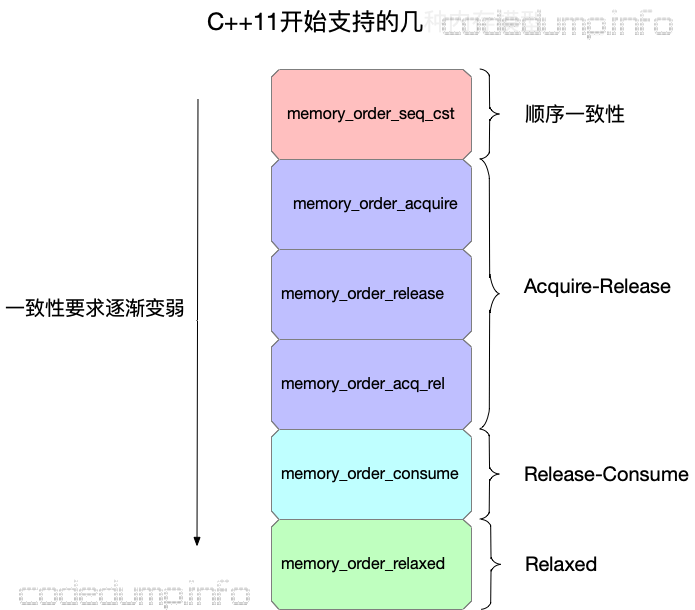# memory_order_seq_cst

#include <atomic>
#include <assert.h>

std::atomic<bool> x,y;
std::atomic<int> z;

void write_x()
{
x.store(true,std::memory_order_seq_cst);
}

void write_y()
{
y.store(true,std::memory_order_seq_cst);
}

{
++z;
}

{
++z;
}

int main()
{
x=false;
y=false;
z=0;
a.join();
b.join();
c.join();
d.join();
}


# memory_order_relaxed

• 针对一个变量的读写操作是原子操作；
• 不同线程之间针对该变量的访问操作先后顺序不能得到保证，即有可能乱序。

#include <atomic>
#include <assert.h>

std::atomic<bool> x,y;
std::atomic<int> z;

void write_x_then_y()
{
x.store(true,std::memory_order_relaxed);
y.store(true,std::memory_order_relaxed);
}

{
++z;
}

int main()
{
x=false;
y=false;
z=0;
a.join();
b.join();
}


# Acquire-Release

• memory_order_acquire：用来修饰一个读操作，表示在本线程中，所有后续的关于此变量的内存操作都必须在本条原子操作完成后执行。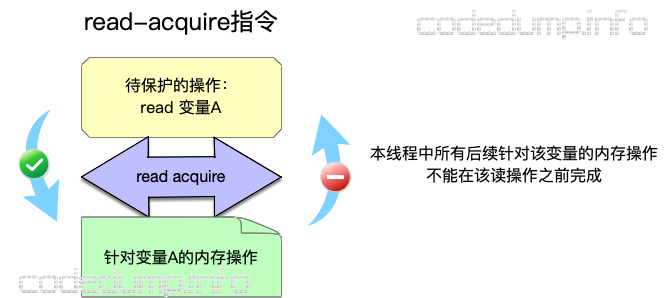• memory_order_release：用来修饰一个写操作，表示在本线程中，所有之前的针对该变量的内存操作完成后才能执行本条原子操作。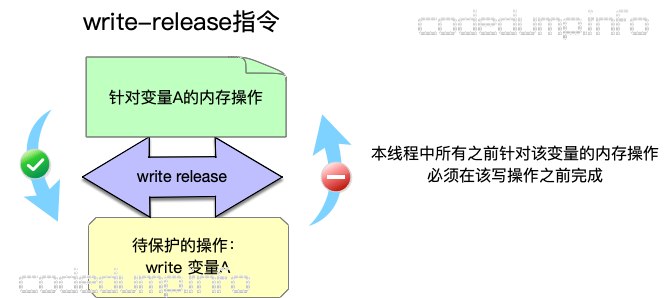• memory_order_acq_rel：同时包含memory_order_acquire和memory_order_release标志。

// 5.7.cpp
#include <atomic>
#include <assert.h>

std::atomic<bool> x,y;
std::atomic<int> z;

void write_x()
{
x.store(true,std::memory_order_release);
}

void write_y()
{
y.store(true,std::memory_order_release);
}

{
++z;
}

{
++z;
}

int main()
{
x=false;
y=false;
z=0;
a.join();
b.join();
c.join();
d.join();
}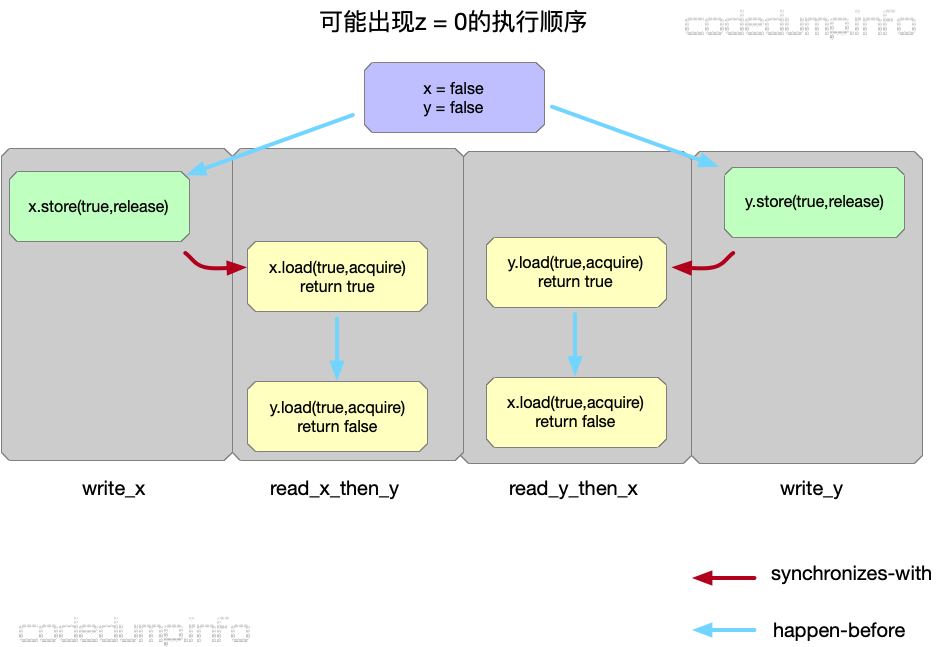• 初始条件为x = y = false。

// 5.8.cpp
#include <atomic>
#include <assert.h>

std::atomic<bool> x,y;
std::atomic<int> z;

void write_x_then_y()
{
x.store(true,std::memory_order_relaxed);
y.store(true,std::memory_order_release);
}

{
++z;
}

int main()
{
x=false;
y=false;
z=0;
a.join();
b.join();
}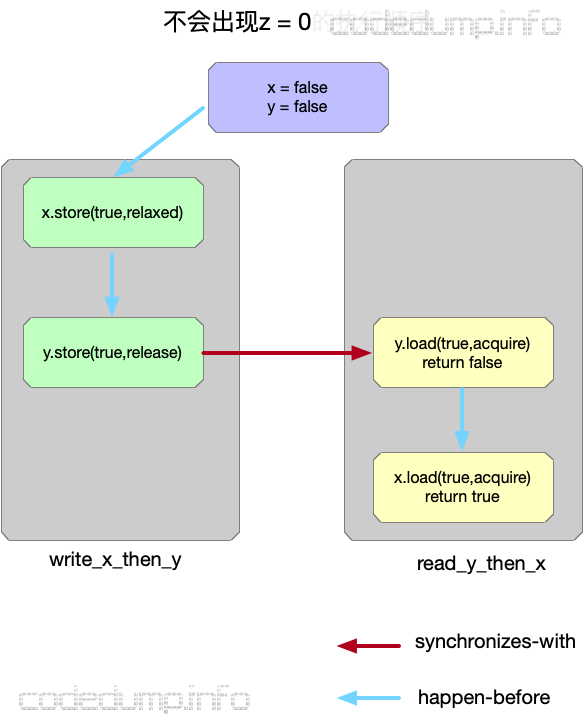• 初始条件为x = y = false。
• 在write_x_then_y线程中，先执行对x的写操作，再执行对y的写操作，由于两者在同一个线程中，所以即便针对x的修改操作使用relaxed模型，修改x也一定在修改y之前执行。
• 因此最终的执行顺序如上图所示，此时不可能出现z=0的情况。

# Release-Consume

b = *a;
c = *b;


// 5.10
#include <string>
#include <atomic>
#include <assert.h>
struct X
{
int i;
std::string s;
};

std::atomic<X*> p;
std::atomic<int> a;

void create_x()
{
X* x=new X;
x->i=42;
x->s="hello";
a.store(99,std::memory_order_relaxed);
p.store(x,std::memory_order_release);
}

void use_x()
{
X* x;
assert(x->i==42);
assert(x->s=="hello");
}
int main()
{
t1.join();
t2.join();
}